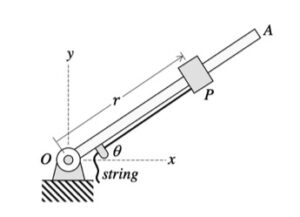# Homework H1.E - Sp23

 Problem statement Solution videoDiscussionAs you watch particle P move through its path, focus on a few things:

• Direction of the velocity vector. The velocity of the ball is always tangent to the path, as expected. Note that the unit tangent vector e_t points in the same direction as the velocity.
• Direction of the acceleration vector. The acceleration of the P always points "inward" on the path, again, as expected. Here you see that the acceleration vector points in the positive e_t direction; therefore, its speed must be increasing with time (as it is).
• Directions of the polar unit vectors. The e_r unit vector always points outward from  point O toward P. The e_θ unit vector is perpendicular to e_r, and in the direction of increasing θ, as seen in the animation above.

Your task is to calculate R and θ, and their time derivatives. Here, you are given R and θ explicitly as a function of time. Therefore, the chain rule of differentiation is not needed.

## 24 thoughts on “Homework H1.E - Sp23”

1.William Grant Dierking says:

Similar to last HW, do we leave our answers in terms of er and eo or convert to cartesian? Thanks

1.Madeline B says:

Since our lecture was based of polar kinematics, my best guess would be to convert to e_r and e_theta.

2.CMK says:

Since the problem does not specify which set of coordinates that are expected for the final answer, you are free to choose either one. My recommendation would be to keep it in terms of the polar unit vectors, as that would be less work for you.

3.Andrew Thomas Zaslavsky says:

We do not have to convert to cartesian, it does not ask to

2.Cael Patrick Fitch says:

Is the problem asking for the scalar magnitude of the velocity and acceleration or the vector form of velocity and acceleration?

1.CMK says:

Velocity and acceleration are vectors. Unless otherwise stated, always give answers for velocity and acceleration as vectors.

2.Andrew Joseph St. Aubin says:

I'm pretty sure they are asking us to find the vectors of velocity and acceleration. They would tell us if they wanted us just to find the scalar of each.

3.Joseph Michael Poetz says:

Were are given a value for t. Does this mean we should give our answers for velocity and acceleration at time=4s or should we keep them as a function of t?

1.Devin Lukas Buergermeister says:

I think we would plug t in

2.Branson Dao says:

Yes--plug in the value t = 4 for the equations you find for the corresponding velocity and acceleration vectors.

3.Andrew Thomas Zaslavsky says:

You should plug in t = 4s into the functions

4.Melissa Jewel Brock says:

Do both components of the vectors have the same units?

1.Branson Dao says:

I believe so? I left them as the same units--I'm thinking it is similar to ( X i + Y j ) unit/second.

2.CMK says:

Yes, they need to be the same. Each component of velocity must have units of m/s. Each component of acceleration must have units of m/s^2. Otherwise, you cannot add together the components (it is an apples-and-oranges thing).

5.Trysta Chiang says:

To do the derivatives of the formula 'r', can we just use the formula given in the question, or do we need to plug it into the formula r-hat = r*er-hat?

1.Trenton James Bien says:

Im pretty sure you first take the derivative, then use the two functions discussed in class to turn it into the forms with er-hat and etheta-hat.

6.Griffin Collier says:

Since we need to get everything into m/s, how do we convert theta and theta_dot given in rad/s into m/s?

7.Elijah Allan Collins says:

Since we are given r and theta in terms of t, can we just take the r_dot and theta_dot to find the components of the velocity vector and then r_dot_dot and theta_dot_dot for the acceleration vectors or am I missing something?

1.Elijah Allan Collins says:

or do we need to use the equations v = r_dot (e_hat_r) + r*theta_dot(e_hat_theta)? And the long formula for the acceleration?

1.Mason James Fellwock says:

My assumption is that we would use the equations. Take the derivative of the given r and theta equations twice, plug in t=4sec and substitute into the equations.

8.Dev Chirag Lekhadia says:

Are the lecture videos posted?

9.Trisha Boodhoo says:

Did anyone else find that acceleration didn't have a tangential component and velocity didn't have an er vector? Since both these unit vectors must be perpendicular it would make sense but, I am concerned that this may be due to improper differentiation.

1.Trevor James Daugerdas says:

I didn't find that, are you using the formula we derived in lecture for velocity and acceleration?

2.Phillip Eugene Holmes says:

If you plugged in the equation for theta and then solved for vbar = rdot * er + r * thetadot * etheta . you would get both er and e theta components for velocity. similarly solving the equation for acceleration would yield both er and etheta components